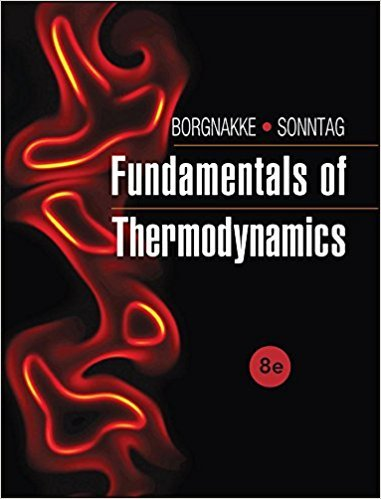×
Get Full Access to Fundamentals Of Thermodynamcs - 8 Edition - Chapter 7 - Problem 21hp
Get Full Access to Fundamentals Of Thermodynamcs - 8 Edition - Chapter 7 - Problem 21hp

×

# A reversible isothermal expander (a turbine with heatISBN: 9781118131992 58

## Solution for problem 21HP Chapter 7

Fundamentals of Thermodynamcs | 8th Edition

• Textbook Solutions
• 2901 Step-by-step solutions solved by professors and subject experts
• Get 24/7 help from StudySoup virtual teaching assistantsFundamentals of Thermodynamcs | 8th Edition

4 5 1 403 Reviews
25
0
Problem 21HP

Problem 21HP

A reversible isothermal expander (a turbine with heat transfer) has an inlet flow of carbon dioxide at 3MPa, 80° C and an exit flow at 1 MPa, 80° C. Find the specific heat transfer from the entropy equation and the specific work from the energy equation assuming ideal gas.

Step-by-Step Solution:
Step 1 of 3

1 Chapter 1: Scientific Measurements Objectives: ­Experiment, law, hypothesis, theory ­Matter ­physical change (property) vs chemical change (property) ­Substances, elements, compounds, mixtures ­Significant figures ­The scientific method ­Classifications of matter ­Measurement ­Density and Specific Gravity ­You must already be familiar with Chapter 1 content. It is considered a self – review chapter. ­Chemistry: cisplatin leading anticancer drug ­Development of life saving drugs ­an intellectual enterprise, a way of explaining our material world ­DNA Scientific method: The systematic approaches which scientists take to answer questions and solve problems (research). Collect information

Step 2 of 3

Step 3 of 3

##### ISBN: 9781118131992

Since the solution to 21HP from 7 chapter was answered, more than 260 students have viewed the full step-by-step answer. This full solution covers the following key subjects: equation, transfer, specific, mpa, heat. This expansive textbook survival guide covers 7 chapters, and 1462 solutions. Fundamentals of Thermodynamcs was written by and is associated to the ISBN: 9781118131992. The answer to “A reversible isothermal expander (a turbine with heat transfer) has an inlet flow of carbon dioxide at 3MPa, 80° C and an exit flow at 1 MPa, 80° C. Find the specific heat transfer from the entropy equation and the specific work from the energy equation assuming ideal gas.” is broken down into a number of easy to follow steps, and 49 words. This textbook survival guide was created for the textbook: Fundamentals of Thermodynamcs , edition: 8. The full step-by-step solution to problem: 21HP from chapter: 7 was answered by , our top Engineering and Tech solution expert on 08/03/17, 05:05AM.

Unlock Textbook Solution